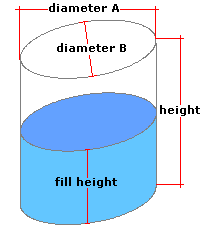Spike's Calculators

# Partial Volume in an Vertical Ellipse Cylinder TankCalculate the amount of liquid in a partially filled vertical elliptical cylinder tank.
This shape of tank/container is like a vertical cylinder, but instead of having a circular base and top, they are in the shape of an ellipse. This calculator uses inches for measurements.

##### Approximate weight of Fuel or Water
```one US gallon of water = 8.34 pounds.
one US gallon of diesel fuel = 7.1 pounds
one US gallon of gas = 6 pounds
```

### Elliptical Cylinder Tank

Diameter A in
Diameter B in
Tank Height in
Depth of Liquid in
Liquid Weight-Pounds per US Gallon lb/gal US

#### Results:

###### Tank Volume and Capacity
In Cubic Inches in³
In Cubic Feet ft³
Cubic Metres
US Gallons gal US
###### Amount of Liquid Inside Tank
In US Gallons gal US
In Imperial Gallons gal Imperial
In Litres L
In Cubic Feet ft³
###### Weight of Liquid and Fill Amount
Weight of Liquid lbs
To Fill this Tank, Add gal US

#### Calculator

1. enter the diameter A length in inches
2. the diameter B length in inches
3. the height of the tank in inches
4. the depth of the liquid inside the tank in inches
5. the weight of the liquid in pounds per US gallon

#### Results

1. the volume of the elliptical cylinder tank in cubic inches
2. the volume of the tank in cubic feet
3. the volume of the tank in cubic metres
4. the total amount of liquid the tank can hold in US gallons
5. the amount of liquid inside the tank in US gallons
6. the amount of liquid inside the tank in Imperial gallons
7. the amount of liquid inside the tank in litres
8. the amount of liquid inside the tank in cubic feet
9. the weight of the liquid inside the tank based on lbs/gal US
10. the amount of liquid it will take to fill this tank in US gallons

##### Formula
```V = 𝝅abh
where V is the volume of the tank
a halve the diameter A
b halve the diameter B
h the height of the liquid inside the tank
```
##### Conversions
```one cubic inch (in³) = 0.000578703703703704 cubic feet (ft³)
one cubic inch (in³) = 0.000016387064 cubic metres (m³)
one cubic inch (in³) = 0.00432900432900432 gallons (gal) US
one cubic inch (in³) = 0.00360465014990905 gallons (gal) Imperial
one cubic inch (in³) = 0.016387064 litres (L)
```

### Ellipse - Vertical Tank/Container#### Filled